Rationals & Irrationals Numbers MathBitsNotebook.comCheck out the subsets of the Real Numbers shown in the diagram at the right. Notice that the rational and irrational numbers are contained within the set of Real Numbers.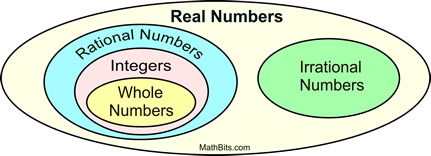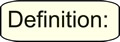A rational number is a number that can be expressed as a fraction (ratio) in the form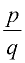where p and q are integers and q is not zero.

Examples: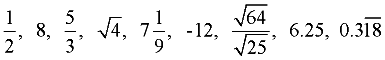A rational number can be expressed as a ratio (fraction).

When a rational number fraction is divided to form a decimal value,
it becomes a terminating or repeating decimal.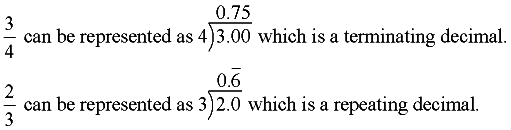Some rational fractions may produce a large number of digits in their repeating patterns, which may exceed the size of the viewing screen on a calculator. The fraction 53/83 has a calculator display of 0.6385542169, which shows no repeating pattern, when in reality the pattern will repeat after 41 digits.To convert a repeating decimal to a fraction: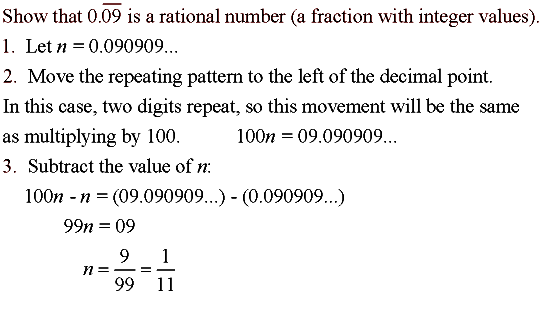To show that the rational numbers are dense:
(between any two rationals there is another rational)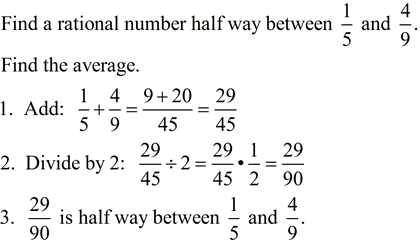An irrational number is a number that is NOT rational. It cannot be expressed as a fraction with integer values in the numerator and denominator.

Examples: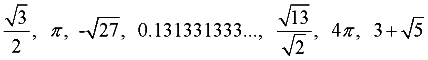When an irrational number is expressed in decimal form, it goes on forever without repeating.

 Regarding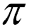: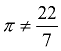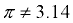While it is popular to use 3.14 or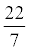to represent "pi", these values are only estimates or approximations. Notice the differences in the decimal representations on the calculator screen at the right.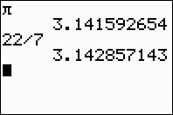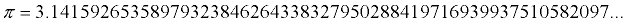Properties of Rational and Irrational Numbers:

Since rational and irrational numbers are subsets of the real numbers,
they possess all of the properties assigned to the real number system.